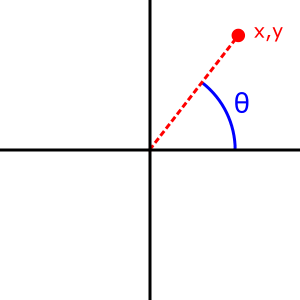/JavaScript

# Math.atan2()

The Math.atan2() function returns the angle in the plane (in radians) between the positive x-axis and the ray from (0,0) to the point (x,y), for Math.atan2(y,x).

## Syntax

Math.atan2(y, x)


### Parameters

y

The y coordinate of the point.

x

The x coordinate of the point

### Return value

The angle in radians (in $\left[-\pi, \pi\right]$) between the positive x-axis and the ray from (0,0) to the point (x,y).

## Description

The Math.atan2() method returns a numeric value between -π and π representing the angle theta of an (x, y) point. This is the counterclockwise angle, measured in radians, between the positive X axis, and the point (x, y). Note that the arguments to this function pass the y-coordinate first and the x-coordinate second.Math.atan2() is passed separate x and y arguments, and Math.atan() is passed the ratio of those two arguments.

Because atan2() is a static method of Math, you always use it as Math.atan2(), rather than as a method of a Math object you created (Math is not a constructor).

## Examples

### Using Math.atan2()

Math.atan2(90, 15); // 1.4056476493802699
Math.atan2(15, 90); // 0.16514867741462683

Math.atan2(±0, -0);               // ±PI.
Math.atan2(±0, +0);               // ±0.
Math.atan2(±0, -x);               // ±PI for x > 0.
Math.atan2(±0, x);                // ±0 for x > 0.
Math.atan2(-y, ±0);               // -PI/2 for y > 0.
Math.atan2(y, ±0);                // PI/2 for y > 0.
Math.atan2(±y, -Infinity);        // ±PI for finite y > 0.
Math.atan2(±y, +Infinity);        // ±0 for finite y > 0.
Math.atan2(±Infinity, x);         // ±PI/2 for finite x.
Math.atan2(±Infinity, -Infinity); // ±3*PI/4.
Math.atan2(±Infinity, +Infinity); // ±PI/4.


## Browser compatibility

Desktop Mobile Server
Chrome Edge Firefox Internet Explorer Opera Safari WebView Android Chrome Android Firefox for Android Opera Android Safari on IOS Samsung Internet Deno Node.js
atan2
1
12
1
4
3
1
1
18
4
10.1
1
1.0
1.0
0.10.0